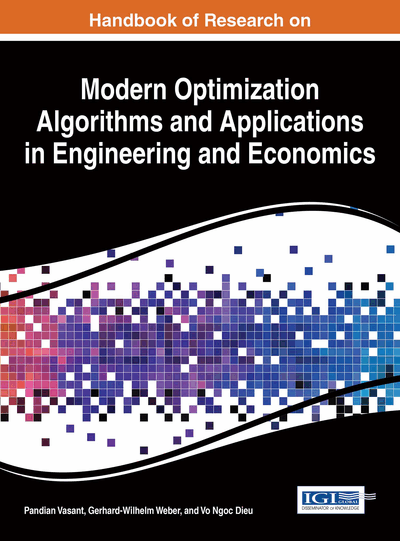# TOPSIS in Generalized Intuitionistic Fuzzy Environment

Amal Kumar Adak (Jafuly Deshpran High School (H.S.), India), Debashree Manna (Damda Jr. High School, India), Monoranjan Bhowmik (Asam Central University (Silchar), India) and Madhumangal Pal (Vidyasagar University, India)
DOI: 10.4018/978-1-4666-9644-0.ch024

## Abstract

The aim of this chapter is to investigate the multiple attribute decision making problems to a selected project with generalized intuitionistic fuzzy information in which the information about weights is completely known and the attributes values are taken from the generalized intuitionistic fuzzy environment. Here, we extend the technique for order performance by similarity to ideal solution (TOPSIS) for the generalized intuitionistic fuzzy data. In addition, obtained the concept of possibility degree of generalized intuitionistic fuzzy numbers and used to solve ranking alternative in multi-attribute decision making problems.
Chapter Preview
Top

## Introduction

A lot of multiple criteria decision making (MCDM) approaches have been developed and applied to diverse fields, like engineering, management, economics etc. As one of the known classical MCDM approaches, TOPSIS (technique for the order preference by similarity to ideal solution) was first developed by Hwang and Yoon (1981). The primary concept of TOPSIS approach is that the most preferred alternative should not only have the shortest distance from the positive ideal solution but also have the farthest distance from the negative ideal solution. The advantages for the TOPSIS include (a) simple, rationally, comprehensive concept, (b) good computational efficient, (c) ability to measure the relative performance for each alternative in a simple mathematical form.

Zadeh (1965) introduced first the theory of fuzzy sets. Later on, many researchers have been working on the process of dealing with fuzzy decision making problems by applying fuzzy set theory. Atanassov (1986) introduced the concept of intuitionistic fuzzy sets (IFSs). IFSs are proposed using two characteristic functions expressing the degree of membership and the degree of non-membership of elements of the universal set to the IFS. Adak (2011b, 2012b)et al. investigated some interesting properties of intuitionistic fuzzy sets and matrices. Mondal and Samanta (2002) defined generalized intuitionistic fuzzy sets (GIFSs). Motivated by the definition of GIFSs, Bhowmik (2008) investigated generalized intuitionistic fuzzy matrices (GIFMs). Generalized intuitionistic fuzzy matrices has been applied in multi-criteria decision making problem including conflicting criteria such as political environment, proximity to markets and customers, quality of life issues, culture issues, etc.

In this chapter, we introduce the concept of generalized intuitionistic fuzzy preference relation and develop the TOPSIS method in multi-criteria decision making problem with generalized intuitionistic fuzzy information.

Top

## Background

Decision-making problems are the process of finding the best option from all of the feasible alternatives. In almost all such problems the multiplicity of criteria for judging the alternatives is pervasive. That is for many such problems, the decision maker wants to solve a multiple criteria decision making (MCDM) problem. Technique for order performance by similarity to ideal solution (TOPSIS), one of the known classical MCDM method, was first developed by Hwang and Yoon  for solving a MCDM problem. It bases upon the concept that the chosen alternative should have the shortest distance from the positive ideal solution (PIS) and the farthest from the negative ideal solution (NIS). In the process of TOPSIS, the performance ratings and the weights of the criteria are given as crisp values.

## Complete Chapter List

Search this Book:
Reset